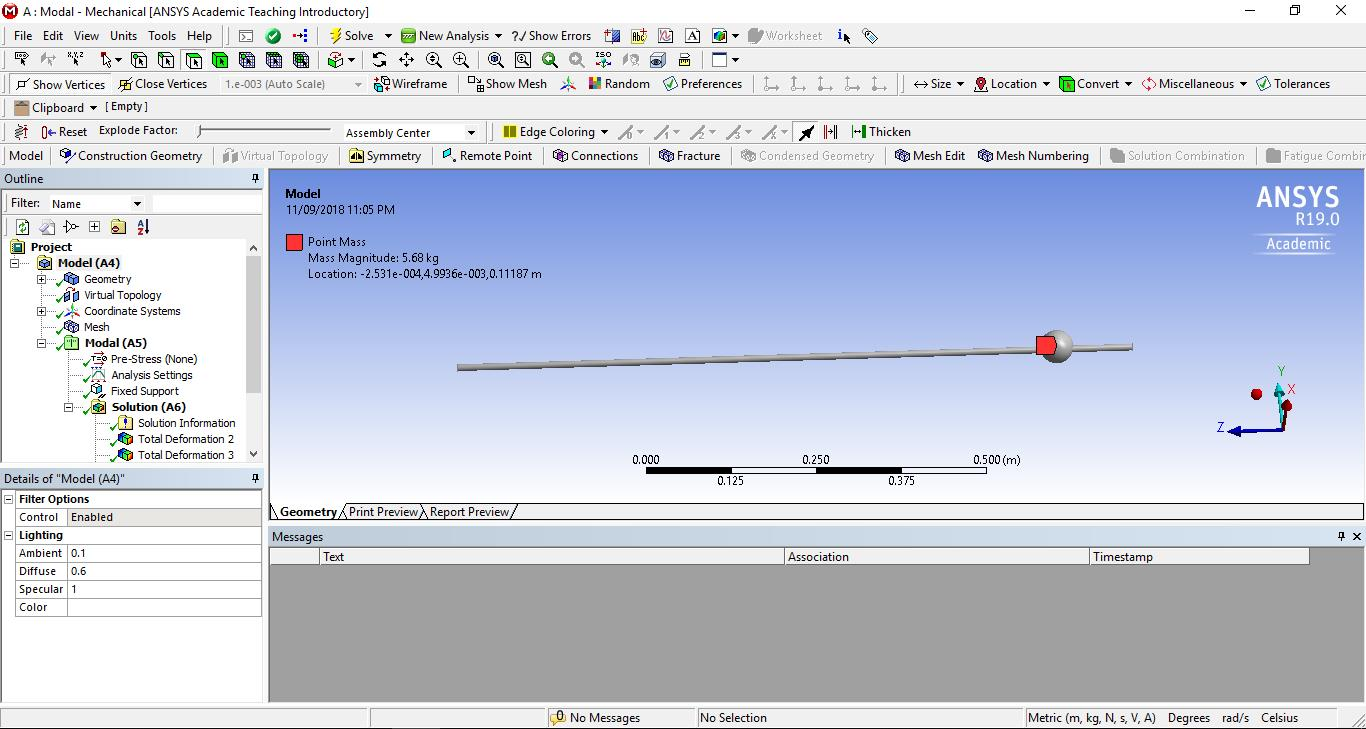## General Mechanical

•o.darwazeh
Subscriber

We are students trying to build a simulation of a translating beam that we can control its velocity and acceleration, and then analyse its data in order to find out the deflection/frequency modes occurred due to the vibration of its movement, mass and gravity.Which Ansys 19.0 product/ analysis system should we be using in order to create this kind of a system and obtain its data and graphs?

And how can we find out a way to build this system since we haven't found any valuable tutorials regarding our system?

Osama Darwazeh

•peteroznewman
Subscriber

Hello Osama,

Let me see if I can describe the project in a more precise way.  Correct me if I got anything wrong.

1) You have a beam that is parallel to a horizontal x-axis.

2) The beam has a uniform cross section.

3) One end of the beam is fixed (cantilevered) from a carriage that moves on a vertical slide, the other end of the beam is free.

4) You can input a velocity or acceleration signal that varies as a function of time to that vertical carriage.

5) There is a point mass on the beam that can be fixed at different locations along the length.

6) You want to predict the vertical displacements along the length of the beam versus time.

7) You want to know the natural frequencies and the mode shapes that can be excited on the beam.

Questions:

A) Will some input signals to the vertical motion of the carriage be a pure sinusoidal signal?Will some input signals to the vertical motion of the carriage be non-periodic?

C) Will the vertical deformation in the beam be limited to displacements of less than 5% of beam length?

You will want to use analysis systems: Modal and either Harmonic Response or Transient Structural depending on your answers to questions ABC.

Regards,

Peter

•o.darwazeh
Subscriber

Hello Peter,

- the carriage moves on a horizontal slide.

A&B no the inputs are neither pure sinusoidal nor periodic.

C the deformation is expected to be less that 5%.

Regards,

Osama

•peteroznewman
Subscriber

Hello Osama,

Because you have non-harmonic input motion you must use Transient Structural to solve for the motion of the beam.

Because you will input the velocity/acceleration of the horizontal carriage, you can't use Modal Superposition, but will do a Full Transient solution.

Regards,

Peter

•o.darwazeh
Subscriber

Hello Peter,

What general steps should we consider to build our model using Transient Structural?

Regards,

Osama

•peteroznewman
Subscriber

Hello Osama,

Use Beam elements for your model, because they will make a very small model compared with shell or solid element models.

Create a Modal analysis system of the model that has a Fixed Support on one end to obtain the Mode shapes and frequencies.

Create a Transient Structural system and put a horizontal translational joint on one end of the beam. Put a Joint Load on that joint with the velocity or acceleration input.

Do an FFT on the time-history input signal to look at the frequency content. The time-history input to the load must have a sample rate high enough to put a minimum of 20 data points per cycle on the highest frequency in the signal. If it doesn't, you may want to filter the signal and use the filtered signal instead of the original signal in the joint load.

Use matlab or some other program to integrate the acceleration signal twice to see what the displacement range is. Measured acceleration can have a small offset. When integrated, that creates enormous displacements. Cleaning up the acceleration-time history can include mean removal, and trend removal so that the displacement-time history vibrates about a zero mean value.  I can help with this if you want to zip your input signal and attach it for me to look at.

In the Analysis Settings, make sure the maximum time step saves at least 20 outputs per cycle of the highest frequency you want to observe in the output.

Put Displacement Probes on several points on your model. For example the free end.  Output the horizontal Directional Displacement so you have a signal to plot to examine the response of one point on your model to compare with the Directional Displacement of the base.

Regards,

Peter